HandsOn 23 - Deriving the Motion of Molecules

In the following exercise you derive the speed of gas molecules and the distance between collisions, two values that were previously given to you. To do this, you may need to review a little Newtonian mechanics and thermodynamics.

1. Speed of gas molecules. The average kinetic energy of a molecule in a gas at absolute temperature T is approximately (3/2)kT, where k is Boltzmann's constant (1.381  x 10-23 joules/degree Kelvin), i.e.,

 3 2 kT = 1 2 mvavg2
(6.2)

From this, find an expression for the average speed vavg of a gas molecule at room temperature (approximately 300 Kelvin).

 Q6.11: Using this expression, first write an expression for vavg. Using this new expression and the mass of an ammonia molecule, 17 .1.66.10-27kg, find the value of vavg.

2. Distance between collisions. A gas molecule will experience a collision if the distance from its center to another molecule is equal to the sum of their two radii, or approximately equal to the diameter d of a single molecule. We can think of a moving molecule as sweeping out a volume in one second which is equal to its velocity v (meters/second) times the area A = A =
d2, where d is this diameter (see Figure 6.5);

 volume second = v A =d2.
(6.3)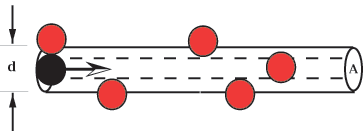Figure 6.5: A moving molecule collides with any other molecule whose center lies within a cylinder of radius equal to the twice the radius of the molecules.

The moving molecule will collide with any other molecule whose center lies in this volume. (Of course whenever the molecule hits another molecule, it changes direction. So the cylinder shown in the figure will be broken up into many short segments. But the volume swept out will still be given approximately by the expression above.)

How many gas molecules are to be found with their centers inside this volume V, on the average? Let the density of gas molecules be n (molecules/meters3). Then the average number of molecules whose centers are to be found in this volume is nV. But the volume V is swept out by the incident particle in one second. Therefore the average number of collisions per second is given by the expression

 (collisions/second) = NV second = nvavgd2.
(6.4)

 Q6.12: From this result and the average speed of the molecule, find an approximate value for the distance Lstep the ammonia molecule travels between collisions. To get n, assuming atmospheric pressure and a temperature of 300 Kelvin, use the gas law equation n = P/kT, which gives approximately 2.4  x 1025 molecules/m3. Finally, for ammonia, the so-called collision diameter d is equal to 3.05  x 10-10m.

 Q6.13: The values for vavg and Lstep you derived here may be different from the values you used to estimate the time t for the ammonia smell to cross the x = 10 centimeter distance. Recalculate the time t using your new values.

Where along the tube will the dust disk appear? It does not appear in the center. Why? Because the ammonia molecules move faster, on the average, than the hydrogen chloride molecules. To see why this is, we need again a little bit of thermodynamics as well as mechanics. The diffusion tube and its contents are at room temperature. This means that the kinetic energies of the ammonia molecules and the hydrogen chloride molecules are, on average, the same. The kinetic energy K of a molecule is given by the expression

 K = 1 2 mv2,
(6.5)
where m is the mass of the molecule and v is its velocity. Since the two gases are at the same temperature, the average kinetic energies of their molecules are equal
 1 2 mHClvHCl2 = 1 2 mNH3vNH32.
(6.6)
From this we conclude that the ratio of velocities is
=
. (6.7)
Suppose (wrongly!) that the ammonia and hydrogen chloride molecules are fired like rifle bullets from opposite ends of the tube. This corresponds to the "ballistic'' setting in the Diffusion Chamber program. Then the distance traveled by each in the time t for them to meet would be given by the expressions
 xNH3 = vNH3(6.8)
and
 xHCl = vHCl.
(6.9)
The ratio of these positions is therefore
==. (6.10)
Now, the ammonia and hydrogen chloride molecules are definitely not fired like rifle bullets from opposite ends of the tube. Instead, these molecules follow a random walk, colliding with air molecules as they diffuse along the tube. Evidence for this conclusion comes from the time required for the powder disk of ammonium chloride to form. The disk would form in a small fraction of a second if the molecules traveled directly. Instead, the time required is many seconds.

In many textbooks, Eq. (10) is used to describe diffusion, even though molecules do not move ballistically-in straight lines-during the diffusion process. These "derivations'' are wrong. However, it sometimes happens in science that a wrong derivation can lead to a correct result. In the present case, Eq. (10) turns out to be correct. This equation is derived form the random walk in HandsOn 25. We will use this result in what follows.

The atomic mass of HCl is 1+35.5 = 36.5 and that of NH3 is 14+3 = 17, approximately half as much. Substituting these values into Eq. (10), we have==
= 1.46. (6.11)

According to this theory, then, the ratio of the distances between the powder disk and the ends of the diffusion unit are approximately 1.5 to one. More careful theory-and experiment-give a smaller value than 1.46. There are several reasons for this. One is that HCl (molecular weight 36.5) has more mass than air molecules O2 (molecular weight 32) and N2 (molecular weight 28). Therefore HCl is not deflected randomly in each collision; it tends to shoulder aside the lighter air molecules and keep going in the same direction as before the collision. Typically more than one collision is required to randomize its direction of motion. In effect, the step length L is made greater (because it goes farther before changing direction significantly). By equation x2avg/L2 = Nsteps, the number of steps is decreased, so the HCl diffuses faster than expected, relative to the lighter molecule NH3.

 Q6.14: Which end will the disk be nearer?

Next: HandsOn 24 - Diffusion Chamber